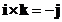## Unit Vector

A unit vector is a vector of unit length . Any non-zero vector can be normalized into a unit vector by a division of a vector by its norm, that is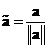.
Note that unit vector is not equal to one vector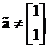, it is the magnitude of the vector is one, not the elements.

## Example:

Suppose we have vector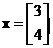The norm of the vector is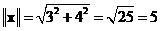Converting it to unit vector becomes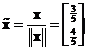Now the norm of the unit vector is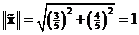The interactive program below will help you to convert your vector input into a unit vector of any positive dimension. The program will also show you the norm of input vector, norm of unit vector (which is always 1) and sum of the unit vector.

Report in rational format

## Properties

Some important properties of unit vector are

• The inner product of a unit vector to itself is one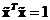.
• Two unit vectors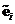and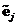are perpendicular to each other (orthogonal) if and only if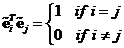.
• In an Euclidean space, the standard unit vectors that orthogonal to each other has name:
• unit vector of the first dimension is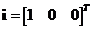• unit vector of the second dimension is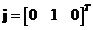• unit vector of the third dimension is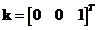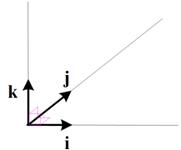• The dot products of the standard unit vector:
• Dot product of the same standard unit vector is one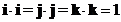• Dot product of the perpendicular standard unit vector is zero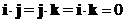• The cross product of the standard unit vectors:
• Cross product of the same standard unit vector is zero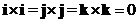• Cross product of the perpendicular standard unit vector form a cycle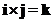;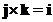;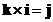;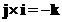;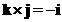;